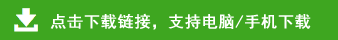# 小学一年级下册数学12套口算题

 29－11= 16－ 4= 89－24= 29－ 8= 98－77= 52－50= 24－13= 10+20= 14+25= 31－11= 17－ 7= 19－12= 98－80= 25－4= 99－23= 24－13= 15+14= 90－10= 20+8= 25+33= 18+11= 28+2= 29+1= 25+25= 26－10= 12+5= 28+11= 25+12= 23－3= 12+10= 16+13= 24+4= 19+11= 17－12= 18－8= 19－6= 29+10= 72－10= 20+30= 99－98= 12+17= 60－40= 17－17= 90－40= 16+54= 20－10= 70－50= 50－30= 60+30= 12+33= 28+6= 16+44= 20+44= 50－50= 19－18= 18－15= 88+12= 66－16= 90－30= 65－25= 50－20= 87－7= 80+9= 18－13= 13+17= 15+53= 16+13= 14+22= 89－80= 14+26= 56－40= 66－20= 99－33= 66－46= 19+60= 17－(  )=0 17－14= 15－3= 80+4= 26－16= 19－(  )=13 27－14= 18+(  )=20 14+(  )=27 67－12= 16－(  )=12 65－33= 65+25= 24+24= 8+(  )=16 10+(  )=50 65+(  )=70 (  )－20=50 40+(  )=60 20+(  )=30 87－33= 16－6= 80－(  )=0 75－25= 30+30=

 69+ 3= 47－ 6= 59+10= 28+ 9= 73+ 6= 4 +50= 22－ 9= 15+ 8= 8 +12= 9 + 6= 18－ 9= 72－ 2= 49+ 7= 27+14= 59－ 6= 82－ 1= 25－ 5= 64+ 5= 89－ 4= 19－ 6= 55+25= 69+13= 48+13= 87－12= 21－ 5= 9 +43= 12+10= 80－20= 67－15= 35－16= 29－13= 66－15= 12－ 7= 10+19= 16－11= 90+10= 29－13= 85－16= 44－13= 22－11= 34+16= 88－18= 60－12= 51－12= 97－17= 17+16= 26+ 3= 67－ 7= 98－18= 29－19= 100－4= 99+ 1= 29－13= 39+ 2= 30+12= 83－11= 50+50= 29－10= 68+ 5= 30+70= 87－10= 77－15= 99－66= 33+11= 16+ 3= 83－19= 26+38= 70+20= 56+16= 60－20= 22+40= 34+12= 13－ 8= 23－ 4= 82－ 6= 82－15= 100－(  )=80 23－(  )=18 44+(  )=60 70－(  )=20 23+16= 55+33= 34+12= 47－30= 21+47= 26+(  )=66 86+9= 66+5= 24+9= 9 +54= 60－16= 16+(  )=56 41－(  )=30 86－12= 73－44= 66+12= 74－15= 69－13= 69+(  )=80 44－15=